# Tiling images with overlap#

When processing images in tiles, we can observe artifacts on borders of the tiles in the resulting image. One strategy to prevent these artifacts is to process tiles with some overlap. dask also supports this. Again, for demonstration purposes, we use imshow to show the resulting images. If these were big data, the imshow function would not work.

import dask
from skimage.filters import gaussian
from skimage.data import cells3d
from pyclesperanto_prototype import imshow


Similar to the example in the last lesson, we define a procedure that applies a Gaussian blur to an image and prints out the size of the image, just so that we know:

def procedure(image):
print("proceduring", image.shape)
return gaussian(image, sigma=5)

image = cells3d()[30,1]
imshow(image)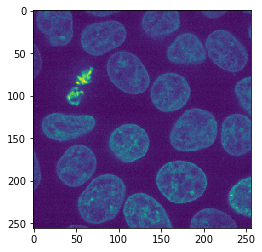tiles = da.from_array(image, chunks=(128, 128))
tiles

 Array Chunk 128.00 kiB 32.00 kiB (256, 256) (128, 128) 4 Tasks 4 Chunks uint16 numpy.ndarray

Next, we tell dask what to do with our tiles: We want to map the function procedure to the tiles with a defined overlap.

overlap_width = 1
tile_map = da.map_overlap(procedure, tiles, depth=overlap_width)

proceduring (0, 0)
proceduring (1, 1)

/Users/haase/opt/anaconda3/envs/bio_39/lib/python3.9/site-packages/dask/array/overlap.py:642: FutureWarning: Default 'boundary' argument value will change from 'reflect' to 'none' in future versions from 2022.03.0 onwards. Use 'boundary="none"' to opt into the future behavior now or set 'boundary="reflect"' to maintain the current behavior going forward.
warnings.warn(


The function was executed twice with very small images (0x0 and 1x1 pixels) to check if it works. Next, we actually compute the result.

result = tile_map.compute() # Warning: This loads all image data into memory

proceduringproceduring (130, 130)
proceduring (130, 130)
(130, 130)
proceduring (130, 130)


From the printed image size, we can see that the processed image size is 2 pixels larger than the tile size. That’s the overlap of 1 pixel in all directions.

## Minimizing border effects#

Next, we will compare the result when processing the whole image with the image processed in tiles with different overlaps. This gives us the chance to figure out the minimum necessary overlap width for eliminating border effects. First, we compute the result for the full image.

untiled_result = procedure(image)

proceduring (256, 256)


Then, we run a for-loop with different border_widths.

for overlap_width in range(0, 25, 5):
print("Overlap width", overlap_width)
tile_map = da.map_overlap(procedure, tiles, depth=overlap_width, boundary='nearest')
result = tile_map.compute()
difference = result - untiled_result
imshow(difference)
print("sum difference", difference.sum())
print("-----------------------------------")

Overlap width 0
proceduring (0, 0)
proceduring (1, 1)
proceduringproceduring (128, 128)
proceduring (128, 128) (128, 128)

proceduring (128, 128)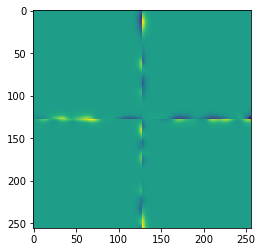sum difference 1.528818863147824
-----------------------------------
Overlap width 5
proceduring (0, 0)
proceduring (1, 1)
proceduring (138, 138)
proceduring (138, 138)
proceduring (138, 138)
proceduring (138, 138)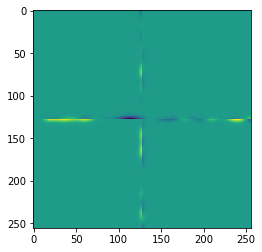sum difference 2.098167990865754
-----------------------------------
Overlap width 10
proceduring (0, 0)
proceduring (1, 1)
proceduringproceduring (148, 148)
(148, 148)
proceduring (148, 148)
proceduring (148, 148)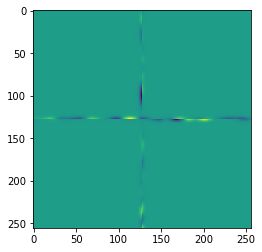sum difference -0.18132395183423158
-----------------------------------
Overlap width 15
proceduring (0, 0)
proceduring (1, 1)
proceduring (158, 158)
proceduring (158, 158)
proceduring (158, 158)
proceduring (158, 158)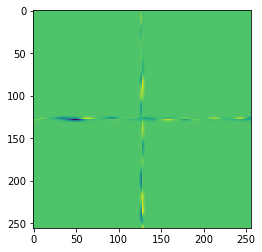sum difference -0.005761703866654207
-----------------------------------
Overlap width 20
proceduring (0, 0)
proceduring (1, 1)
proceduring (168, 168)
proceduring (168, 168)
proceduring (168, 168)
proceduring (168, 168)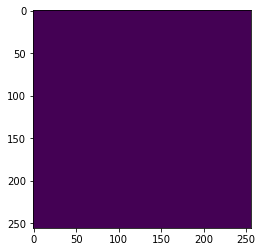sum difference 0.0
-----------------------------------


As you can see, for completely eliminating the border effect, we need to use an overlap of 25 pixels. This is obviously related to the procedure we applied. In our case, the Gaussian blur used in procedure was configured with sigma=5. As a rule of thumb we can say that in the case of a Gaussian blur, the border width must be at least four times the configured sigma. However, when using more complicated algorithms, there are no such rules. In general, it is recommended to test tiled image processing on small images as demonstrated here and figure out if artifacts appear and what error they may cause in a longer image processing workflow.